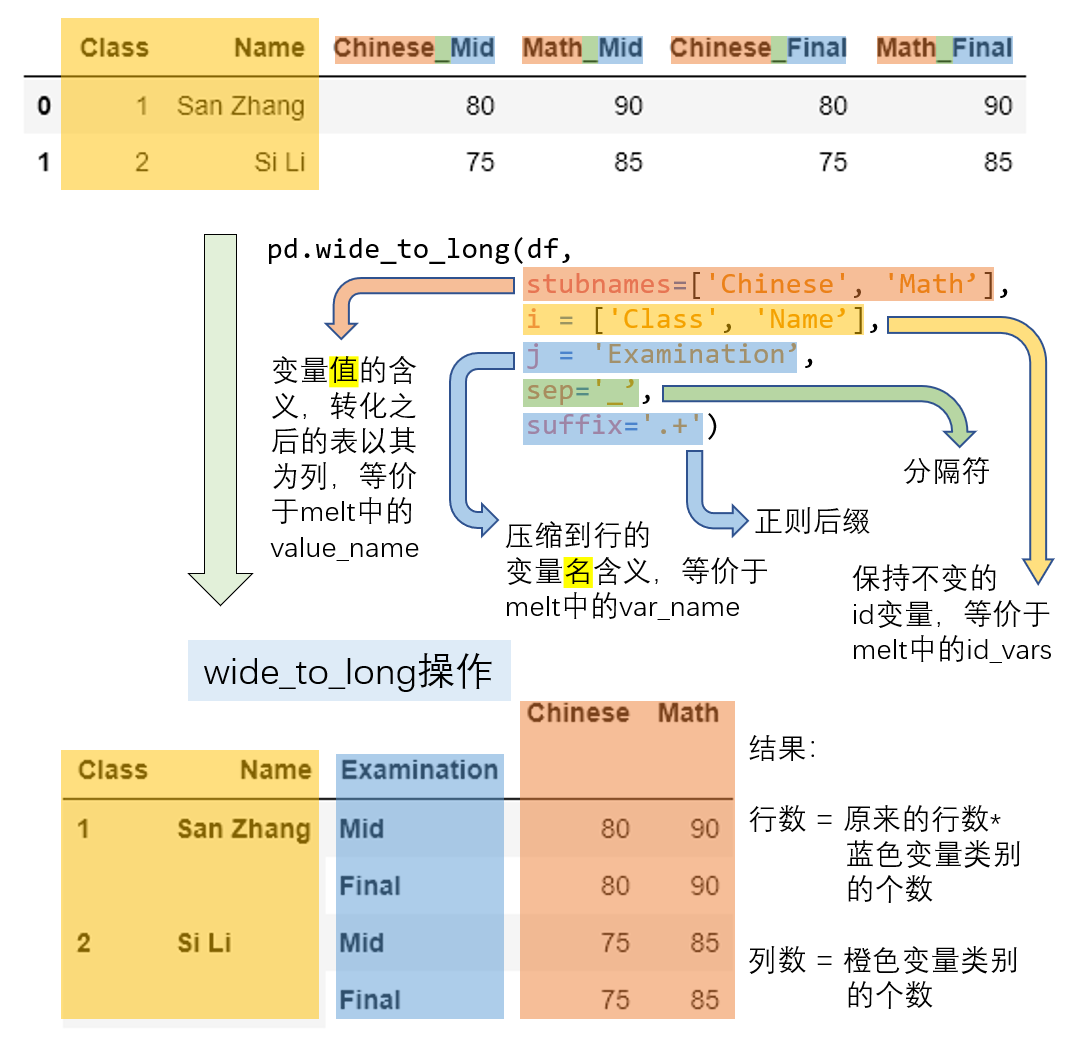signed

QiShunwang

“诚信为本、客户至上”

# pandas基础--变形

2020/12/27 22:27:39   来源：

# 一、长宽表的变形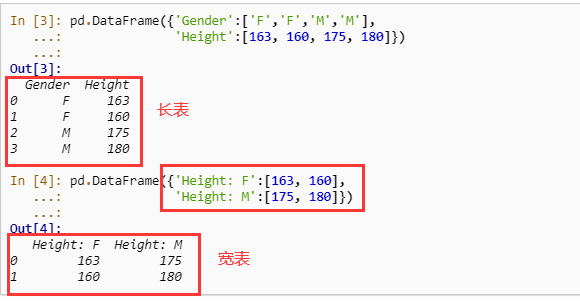## 1. pivot

• pivot 是一种典型的长表变宽表的函数，
• 对于一个基本的长变宽的操作而言，最重要的有三个要素，分别是变形后的行索引、需要转到列索引的列，以及这些列和行索引对应的数值，它们分别对应了`pivot`方法中的`index, columns, values`参数。新生成表的列索引是`columns`对应列的`unique`值，而新表的行索引是`index`对应列的`unique`值，而`values`对应了想要展示的数值列。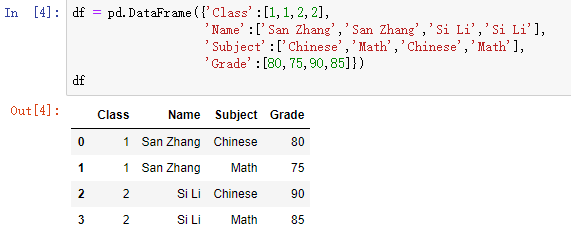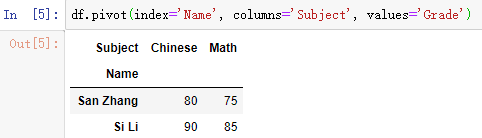• 利用`pivot`进行变形操作需要满足唯一性的要求，即由于在新表中的行列索引对应了唯一的`value`，因此原表中的`index``columns`对应两个列的行组合必须唯一。
• 例如，现在把原表中第二行张三的数学改为语文就会报错，这是由于`Name``Subject`的组合中两次出现`("San Zhang", "Chinese")`，从而最后不能够确定到底变形后应该是填写80分还是75分。
• `pandas``1.1.0`开始，`pivot`相关的三个参数允许被设置为列表，这也意味着会返回多级索引。这里构造一个相应的例子来说明如何使用：下表中六列分别为班级、姓名、测试类型（期中考试和期末考试）、科目、成绩、排名。
``````df = pd.DataFrame({'Class':[1, 1, 2, 2, 1, 1, 2, 2],
'Name':['San Zhang', 'San Zhang', 'Si Li', 'Si Li',
'San Zhang', 'San Zhang', 'Si Li', 'Si Li'],
'Examination': ['Mid', 'Final', 'Mid', 'Final',
'Mid', 'Final', 'Mid', 'Final'],
'Subject':['Chinese', 'Chinese', 'Chinese', 'Chinese',
'Math', 'Math', 'Math', 'Math'],
'Grade':[80, 75, 85, 65, 90, 85, 92, 88],
'rank':[10, 15, 21, 15, 20, 7, 6, 2]})
df
``````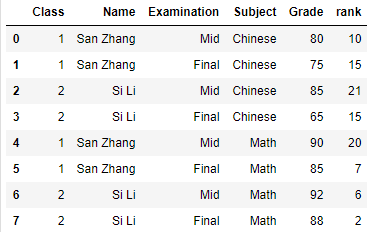• 现在想要把测试类型和科目联合组成的四个类别（期中语文、期末语文、期中数学、期末数学）转到列索引，并且同时统计成绩和排名：
``````pivot_multi = df.pivot(index = ['Class', 'Name'],
columns = ['Subject','Examination'],
pivot_multi
``````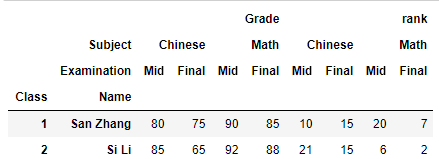• 根据唯一性原则，新表的行索引等价于对`index`中的多列使用`drop_duplicates`，而列索引的长度为`values`中的元素个数乘以`columns`的唯一组合数量（与`index`类似） 。

## 2. pivot_table

• `pivot`的使用依赖于唯一性条件，那如果不满足唯一性条件，那么必须通过聚合操作使得相同行列组合对应的多个值变为一个值。
• 例如，张三和李四都参加了两次语文考试和数学考试，按照学院规定，最后的成绩是两次考试分数的平均值，此时就无法通过`pivot`函数来完成。
``````df = pd.DataFrame({'Name':['San Zhang', 'San Zhang',
'San Zhang', 'San Zhang',
'Si Li', 'Si Li', 'Si Li', 'Si Li'],
'Subject':['Chinese', 'Chinese', 'Math', 'Math',
'Chinese', 'Chinese', 'Math', 'Math'],
'Grade':[80, 90, 100, 90, 70, 80, 85, 95]})
df
``````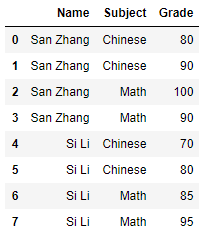• `pandas`中提供了`pivot_table`来实现，其中的`aggfunc`参数就是使用的聚合函数。上述场景可以如下写出：
``````df.pivot_table(index = 'Name',
columns = 'Subject',
aggfunc = 'mean')
``````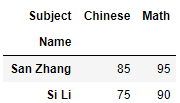• 此外还可以传入以序列为输入标量为输出的聚合函数来实现自定义操作，上述功能可以等价写出：
``````df.pivot_table(index = 'Name',
columns = 'Subject',
aggfunc = lambda x:x.mean())
``````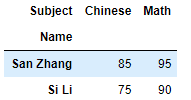• 此外，`pivot_table`具有边际汇总的功能，可以通过设置`margins=True`来实现，其中边际的聚合方式与`aggfunc`中给出的聚合方法一致。下面就分别统计了语文均分和数学均分、张三均分和李四均分，以及总体所有分数的均分：
``````df.pivot_table(index = 'Name',
columns = 'Subject',
aggfunc='mean',
margins=True)
``````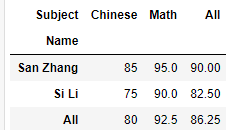## 3. melt

• 长宽表只是数据呈现方式的差异，但其包含的信息量是等价的，前面提到了利用`pivot`把长表转为宽表，那么就可以通过相应的逆操作把宽表转为长表，`melt`函数就起到了这样的作用。在下面的例子中，`Subject`以列索引的形式存储，现在想要将其压缩到一个列中。
``````df = pd.DataFrame({'Class':[1,2],
'Name':['San Zhang', 'Si Li'],
'Chinese':[80, 90],
'Math':[80, 75]})
df
``````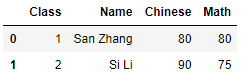``````df_melted = df.melt(id_vars = ['Class', 'Name'],
value_vars = ['Chinese', 'Math'],
var_name = 'Subject',
df_melted
``````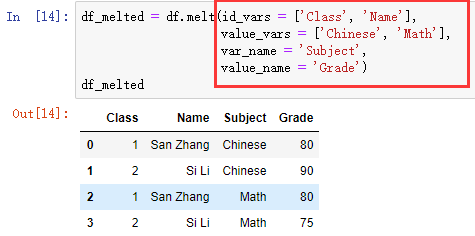• `melt`的主要参数和压缩的过程如下图所示：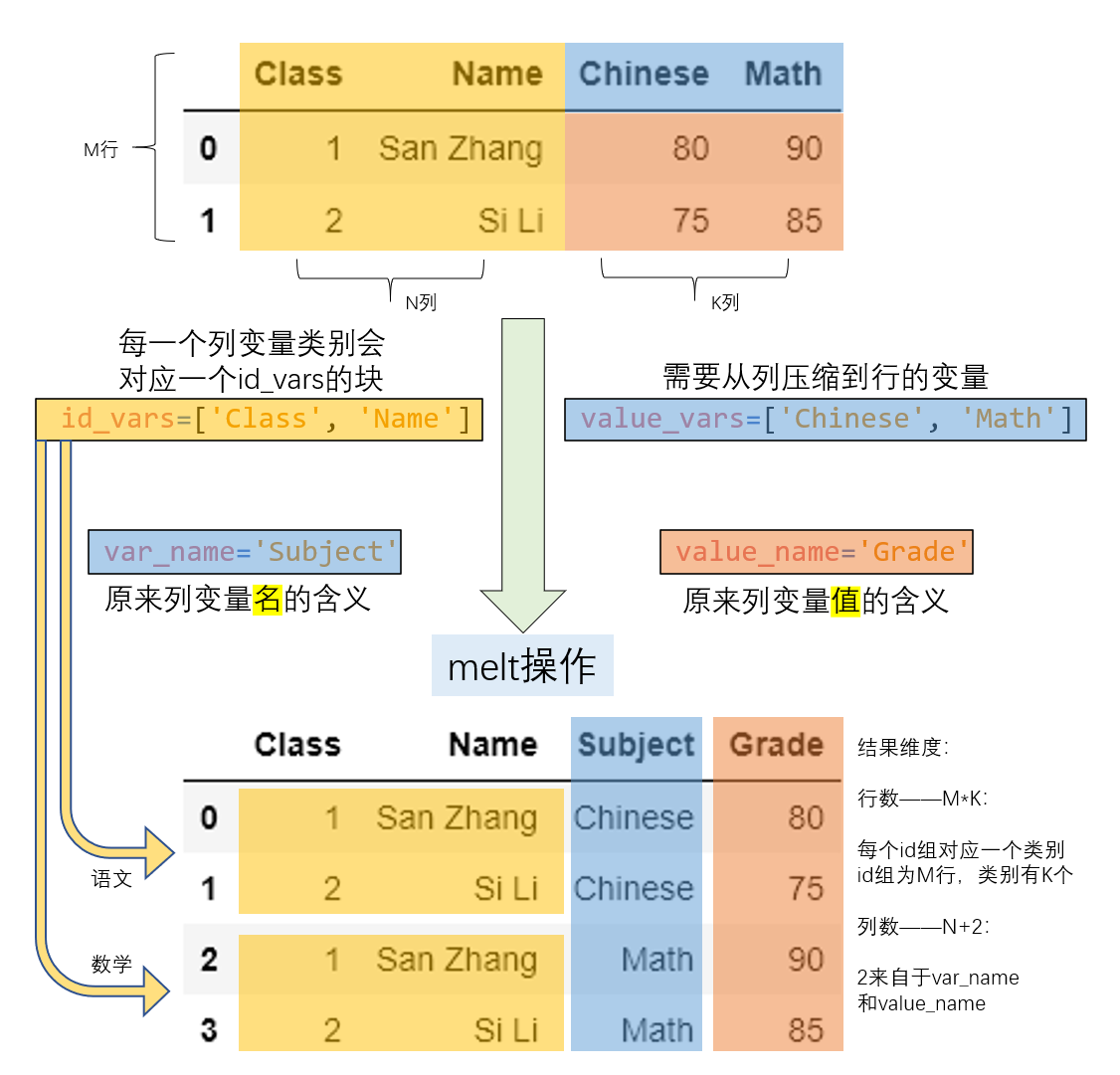• `melt``pivot`是一组互逆过程，那么就一定可以通过`pivot`操作把`df_melted`转回`df`的形式：
``````df_unmelted = df_melted.pivot(index = ['Class', 'Name'],
columns='Subject',
df_unmelted
``````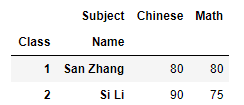## 4. wide_to_long

• `melt`方法中，在列索引中被压缩的一组值对应的列元素只能代表同一层次的含义，即`values_name`。现在如果列中包含了交叉类别，比如期中期末的类别和语文数学的类别，那么想要把`values_name`对应的`Grade`扩充为两列分别对应语文分数和数学分数，只把期中期末的信息压缩，这种需求下就要使用`wide_to_long`函数来完成。
``````df = pd.DataFrame({'Class':[1,2],'Name':['San Zhang', 'Si Li'],
'Chinese_Mid':[80, 75], 'Math_Mid':[90, 85],
'Chinese_Final':[80, 75], 'Math_Final':[90, 85]})
df
``````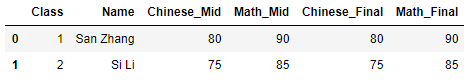``````pd.wide_to_long(df,
stubnames=['Chinese', 'Math'],
i = ['Class', 'Name'],
j='Examination',
sep='_',
suffix='.+')
``````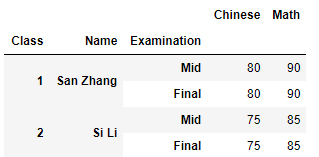• 具体的变换过程由下图进行展示，属相同概念的元素使用了一致的颜色标出：